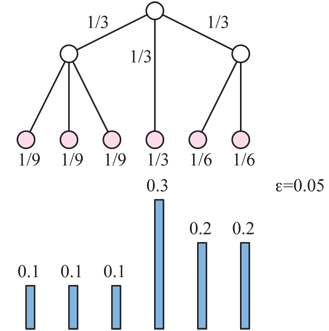# Designing a tree to match a distribution

I want to design a tree to approximate a given sequence of numbers, in the following sense. Let $X=(x_1,\ldots,x_n)$ be $n$ numbers, with $0 < x_i \le 1$ and $\sum_i x_1 = 1$. For a rooted tree $T$, imagine particles traveling from the root to the leaves, branching with equal probability at each junction. So, at a node with $k$ children, $1/k$ of the particles flow to each child. Each leaf then gets assigned the fraction of particles that will accummulate there, multiplying the probabilities at each node on the path from the root to that leaf.

Given $X$, I would like to design a tree $T$ that approximates $X$ within $\epsilon$, meaning that the probabilities at the leaves read left-to-right are each within $\pm \epsilon$ of the corresponding $x_i$. For example, with $X = ( 0.1, 0.1, 0.1, 0.3, 0.2, 0.2 )$ and $\epsilon=0.05$, this tree suffices:In particular, I would like to minimize the number of junctions in $T$:

Given $X$ and $\epsilon$, construct a tree $T$ with as few nodes as possible whose $n$ ordered leaf-probabilities are within $\epsilon$ of the $x_i$.

It feels like this should be polynomial-time rather than intractible, but I am not seeing a clear algorithm. It also feels like it might be a better-known problem in disguise... Any ideas?

(Motivation partially derives from an earlier MO question, Ping-pong relief map of a given function $z=f(x,y)$.)

• Not an answer, but: how DO you get these really cool pictures? Dec 16, 2011 at 20:12
• About the question: I assume by "a tree" you mean that the tree is not necessarily regular, but the probability of going to each child is the same. Is this correct? Dec 16, 2011 at 20:21
• @Igor: Yes, the probability of trickling down to each child is the same. Re pictures: It's not skill; it's love. :-) Dec 16, 2011 at 23:08

Not that it solves the question, but look at the case $n=2$, $x_1=1/3$ and $x_2=2/3$. Not much choice, the only available tree has one internal node and two leaves, so you get the approximation $(1/2,1/2)$ which fails your goal as soon as $\varepsilon<1/6$ ...

One possible remedy is to declare that several of the leave will be "binned" into one outcome - in my example you would get a tree with $3$ leaves as the natural solution, and the last two would map to one state.

Then, a naïve algorithm to produce a working tree would be like this: choose $N$ such that $2^{-N}<\varepsilon$, start with a binary tree of $n$ generations, grouping $\lfloor 2^N x_k \rfloor$ leaves for the $k$-th state, and spread the remaining leaves into the states, at most one per state (which can always be done). You can then prune the tree to reduce depth, if all the leaves below a given node land into the same state.

Is that the kind of construction you had in mind ?

• Excellent point that not all distributions are approximable! Dec 16, 2011 at 23:11
• Fundamentally the problem here is that there is one large $x_i$. Is it the case that all distributions with a small maximum probability are approximable to within a certain error? Obviously true for linear error. Can you do better? Dec 17, 2011 at 5:09

It seems that this: http://en.wikipedia.org/wiki/Chow-Liu_tree is the way to go about it...

• Chow-Liu trees are new to me. I haven't penetrated it completely, but this may be a direct hit. Thanks, Igor! Dec 16, 2011 at 23:10